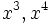# Power-associative magma

This article defines a property that can be evaluated for a magma, and is invariant under isomorphisms of magmas.
View other such properties
This is a variation of semigroup|Find other variations of semigroup |

## Definition

### In terms of the existence of powers

Suppose$(S,*)$ is a magma, i.e.,$S$ is a set and$*$ is a binary operation on$S$. We say that$(S,*)$ is power-associative (or equivalently, that$*$ is power-associative on$S$) if we can define a power function:$S \times \mathbb{N} \to S$

denoted by$(x,n) \mapsto x^n$, such that:$x^1 = x \ \forall x \in S$

and$\! x^m * x^n = x^{m + n} \ \forall x \in S, m,n \in \mathbb{N}$.

If such a function exists, it is unique and$x^n$ equals the value of any$n$-fold product of$x$, no matter how it is parenthesized.

### Other definitions

A power-associative magma is a magma satisfying the following equivalent conditions:

1. It is expressible as the union of subsemigroups, i.e., submagmas that are associative under the operation.
2. It is expressible as the union of abelian subsemigroups, i.e., submagmas that are associative and commutative under the operation.

## Relation with other properties

### Stronger properties

Property Meaning Proof of implication Proof of strictness (reverse implication failure) Intermediate notions
magma in which cubes and fourth powers are well-defined$x^3, x^4$ both make unambiguous sense for all$x$ Magma in which powers up to the fifth are well-defined|FULL LIST, MORE INFO
magma in which powers up to the fifth are well-defined$x^3, x^4, x^5$ are well-defined |FULL LIST, MORE INFO